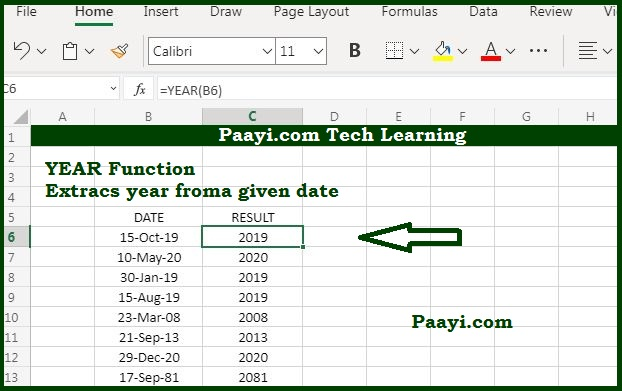# Learn How to Use Microsoft Excel YEAR Function

Written by | 0 Comments | 706 Views

In this article, you will learn how to use the Microsoft Excel YEAR function and its prime function in Microsoft Excel. You will also get to know the Microsoft Excel YEAR function return value and syntax with the help of some examples.

## Microsoft Excel YEAR Function

The primary purpose of the MS Excel YEAR function is to get a year from a specified date. So with the help of the YEAR function, you can able to return the year component of a date in the form of a four-digit number. You can also use the YEAR function to find out the year number from a specific date in a cell or to extract and feed a year value into another formula, such as the DATE function.

### YEAR Function Return Value

The return value will be the four-digit number representing a year.

### YEAR Function Syntax

= YEAR(date)

Where the arguments:

• date: It is the valid Excel date.

## How to Use the Microsoft Excel Year Function?as we know, the YEAR function extracts a year component in the form of a four-digit return value from a specified date; let's understand this by the examples given below:

• = YEAR("17-Sep-2020") - This will return the value 2020.
• = YEAR("26-Jan-2021") - This will return the value 2021.

You can also use the YEAR function to extract a month number from a date in the cell or to feed the month number into another function, as the DATE function. Let us understand this by the example given below:

• =DATE(YEAR(A1),1,1) - This will return first of the same year.

It should be noted that Excel only supported the date begin on January 1, 1990. The date after that is not supported.